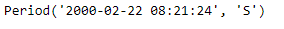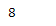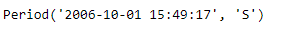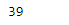Open in App
Not now

# Python | Pandas Period.week

• Last Updated : 06 Jan, 2019

Python is a great language for doing data analysis, primarily because of the fantastic ecosystem of data-centric python packages. Pandas is one of those packages and makes importing and analyzing data much easier.

Pandas` Period.week` attribute returns an integer value which represents the week number in which the given period lies.

Syntax : Period.week

Parameters : None

Return : week of the year

Example #1: Use `Period.week` attribute to find out the week in which the given period object would lie.

 `# importing pandas as pd``import` `pandas as pd`` ` `# Create the Period object``prd ``=` `pd.Period(freq ``=``'S'``, year ``=` `2000``, month ``=` `2``, day ``=` `22``, ``                        ``hour ``=` `8``, minute ``=` `21``, second ``=` `24``)`` ` `# Print the Period object``print``(prd)`

Output :Now we will use the `Period.week` attribute to find the week in which prd object lies.

 `# return the week value``prd.week`

Output :As we can see in the output, the `Period.week `attribute has returned 8 indicating the following period object lies in the 8th week of the given year.

Example #2: Use `Period.week` attribute to find out the week in which the given period object would lie.

 `# importing pandas as pd``import` `pandas as pd`` ` `# Create the Period object``prd ``=` `pd.Period(freq ``=``'S'``, year ``=` `2006``, month ``=` `10``,``               ``hour ``=` `15``, minute ``=` `49``, second ``=` `17``)`` ` `# Print the object``print``(prd)`

Output :Now we will use the `Period.week` attribute to find the week in which prd object lies.

 `# return the week value``prd.week`

Output :As we can see in the output, the `Period.week `attribute has returned 39 indicating the following period object lies in the 39th week of the given year.

My Personal Notes arrow_drop_up